# 标识符的作用域

• 函数原型作用域
• 局部作用域（块作用域）
• 类作用域
• 命名空间作用域

### 1. 函数原型作用域

	double area（double radius）；//函数声明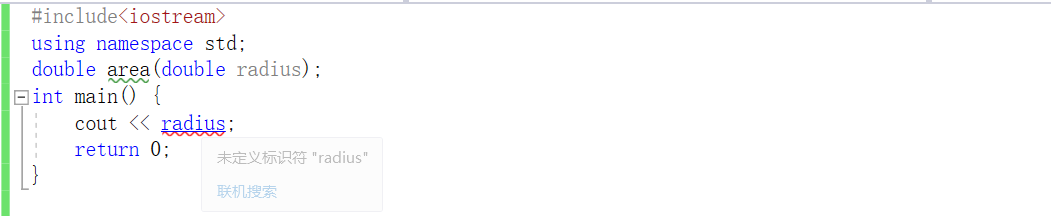### 2.局部作用域(局部变量)

		#include<iostream>
using namespace std;

void fun(int para) {	//定义一个测试函数，para为形参，作用域到该函数大括号为止
cout << "para = " << para<<endl;//可以打印出para = 0
int test1 = 1;		//定义test1，作用域从此到所在块大括号为止
if (test1) {
cout << "--------大括号开始" << endl;
int test2 = 2;		//定义test2，作用域是从此到所在块大括号为止
cout << "test1 = " << test1 << endl;   //在test1作用域范围内，所以可以访问
cout << "test2 = " << test2 << endl;
cout << "--------大括号结束" << endl;
}
cout << "test1 = " << test1 << endl;
cout << "para = " << para << endl;	//分别可以打印出test1 和 para，理解作用域

}
//测试函数
int main() {
fun(0);
return 0;
}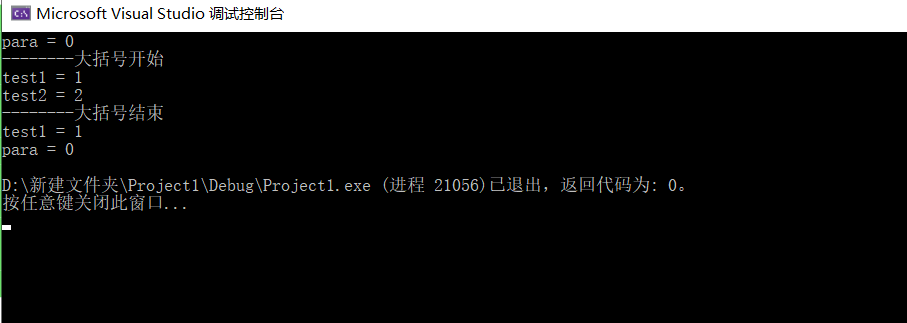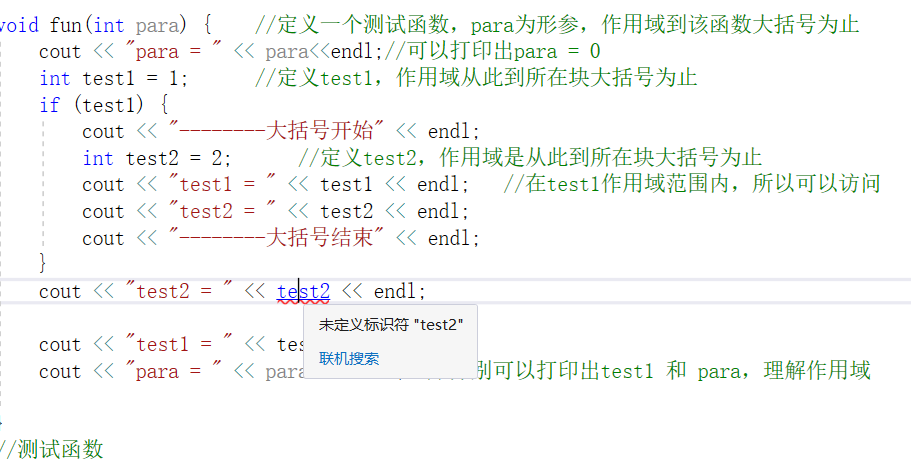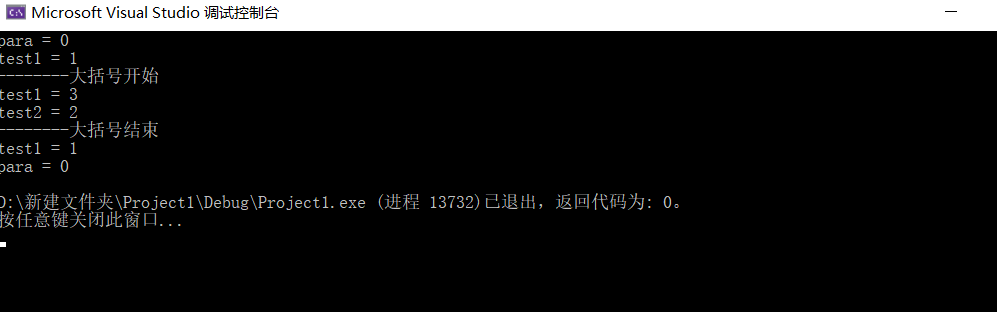• 防止数据被污染。如果不想if里的数据被外部使用，可以在if里面定义，这样在if外部访问会报错。
• 节省内存空间。具有局部作用域的变量也成局部变量，局部变量使用完后会被销毁，腾出空间。

### 3.类作用域

• 若果X的成员函数没有声明同名的局部作用域标识符，那么可以直接访问成员m。若果有同名，可以通过this->m访问。
• x.m 或者 X::m访问，X::m用于访问静态成员。
• ptr->m访问,其中ptr是指向一个类的指针。

		#include<iostream>
using namespace std;

class X {  //定义一个类X
public:
void fun1();
void fun2(int m);
private:
int m = 3;
};
void X::fun1() {
cout << "m =" << m << endl;//没有同名标识符，直接访问
}
void X::fun2(int m) {  //通过X::fun2访问
cout << "传入的m = " << m << endl;  //有同名标识符,打印传入的参数
cout << "访问类的m = " << this->m << endl;
}
int main() {
X x;
x.fun1();  //!!没有参数也要加括号
x.fun2(6);
cout << "指针访问-----------------" << endl;
X* ptr = &x; //给指针赋初值
ptr->fun1();
ptr->fun2(5);
return 0;
}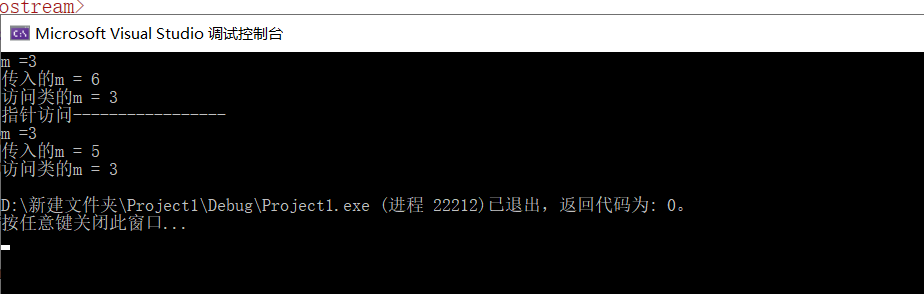### 4.命名空间作用域(全局变量)

	namespace 命名空间名{     //定义

命名空间的声明（函数声明，类申明，全局的数据......）

}
命名空间名::标识符号      /使用

//在另一个作用域使用，c++提供了using语句
using 命名空间名::标识符号;	   //暴露指定标识符
using namespace 命名空间名;    //暴露该命名空间所有标识符


namespace.h

	#pragma once  /*表示该文件只运行一次，，因为文件中如果加载了很多东西，每次需要的时候都要加载的话会浪费运行速度。*/
#include<iostream>
using namespace std;
namespace mynamespace {
class Tangle {
private:
double lon, wid; //只是外部不能访问，可以进行修改
public:
void caltangle(int lon = 1, int wid = 1); //声明caltangle函数，并给lon wid 默认参数
};
}
#endif


namespace.cpp

	#include"./namespace.h"//引入头文件
#include<iostream>

using namespace std;
using namespace mynamespace;//暴露mynamespace所有标识符
//实现caltangle函数，计算长方形的面积
void Tangle::caltangle(int lon=1, int wid=1) {
long area = lon * wid;
cout << "arae = " << area;
}


main.cpp

	#include<iostream>
#include<cmath>
using namespace std;

#include"./namespace.h"  //引入头文件
using namespace mynamespace;  //暴露mynamespace所有标识符
//测试
int main() {
Tangle tag;
int lon, wid;
cout << "enter two number:";
cin >> lon >> wid;
tag.caltangle(lon, wid);
return 0;
}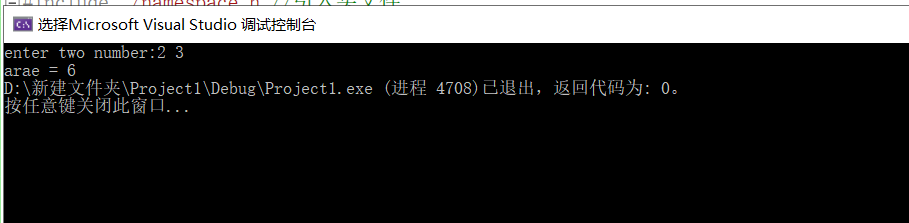posted on 2019-09-26 18:05  SailorMoon-z  阅读(533)  评论(0编辑  收藏  举报